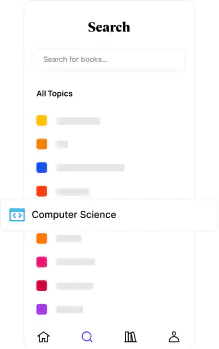📖[PDF] Modern Nonlinear Equations by Thomas L. Saaty | Perlego
###### The online library for learning
Read this book and thousands more for a fair monthly price.📖 Book - PDF

# Modern Nonlinear Equations

Thomas L. SaatyShare book496 pagesEnglishePUB (mobile friendly) and PDFAvailable on iOS & Android
📖 Book - PDF

# Modern Nonlinear Equations

Thomas L. Saaty
Book details

Nonlinear equations have existed for hundreds of years; their systematic study, however, is a relatively recent phenomenon. This volume, together with its companion, Nonlinear Mathematics (Dover 64233-X), provides exceptionally comprehensive coverage of this recently formed area of study. It encompasses both older and more recent developments in the field of equations, with particular emphasis on nonlinear equations because, as Professor Saaty, maintains, `that is what is needed today.`
Together the two volumes cover all the major types of classical equations (except partial differential equations, which require a separate volume). This volume includes material on seven types: operator equations, functional equations, difference equations, delay-differential equations, integral equations, integro-differential equations and stochastic differential equations. Special emphasis is placed on linear and nonlinear equations in function spaces and on general methods of solving different types of such equations.
Above all, this book is practical. It reviews the variety of existing types of equations and provides methods for their solution. It is meant to help the reader acquire new methods for formulating problems. Its clear organization and copious references make it suitable for graduate students as well as scientists, technologists and mathematicians.
`...a welcome contribution to the existing literature...` — Math. Reviews.

## Information

Publisher
Dover Publications
Year
2012
ISBN
9780486143767
Topic
Mathematics
Subtopic
Differential Equations